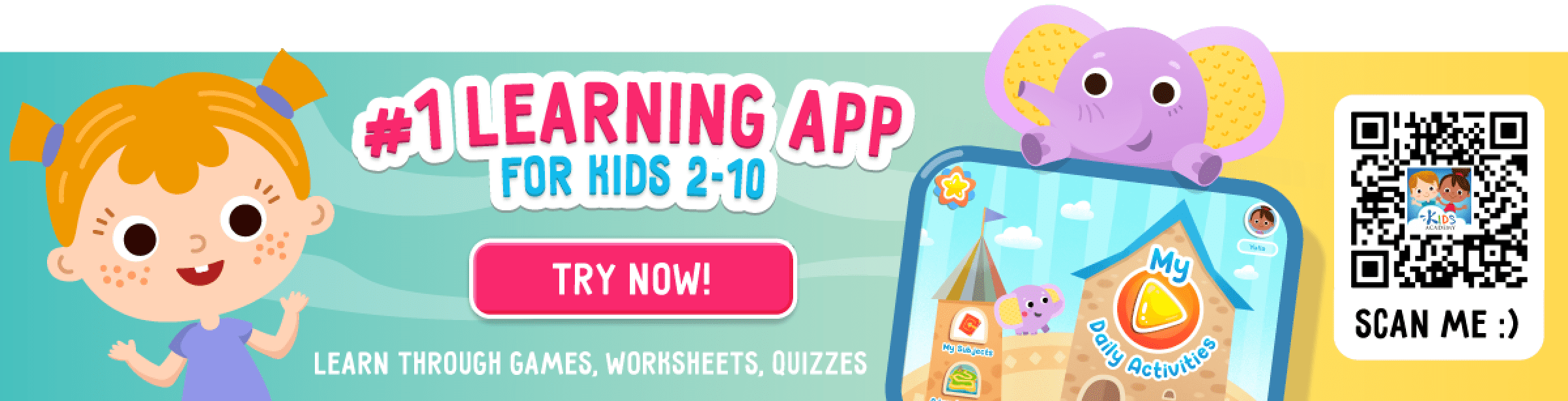# Extra Challenge Subtraction Worksheets

19 results

## Subtraction

Our Extra Challenge Subtraction Worksheets are the perfect choice for preschool to G3 level students. They take the basics of subtraction and give your students an extra push towards subtraction proficiency. Stimulating and engaging, each activity gives your students valuable practice subtracting numbers within 10. With these Subtraction Worksheets, each student will be able to master the basic concepts of subtraction at their own pace. Students are challenged with subtracting the numbers within the frames and will learn the fundamentals of three-digit subtraction. In addition to practice problems, many of our Extra Challenge Subtraction Worksheets include interactive activities and games to keep learning fun and engaging. Get ready to take your students' subtraction skills to the next level!

• Subtraction
• Extra Challenge
Favorites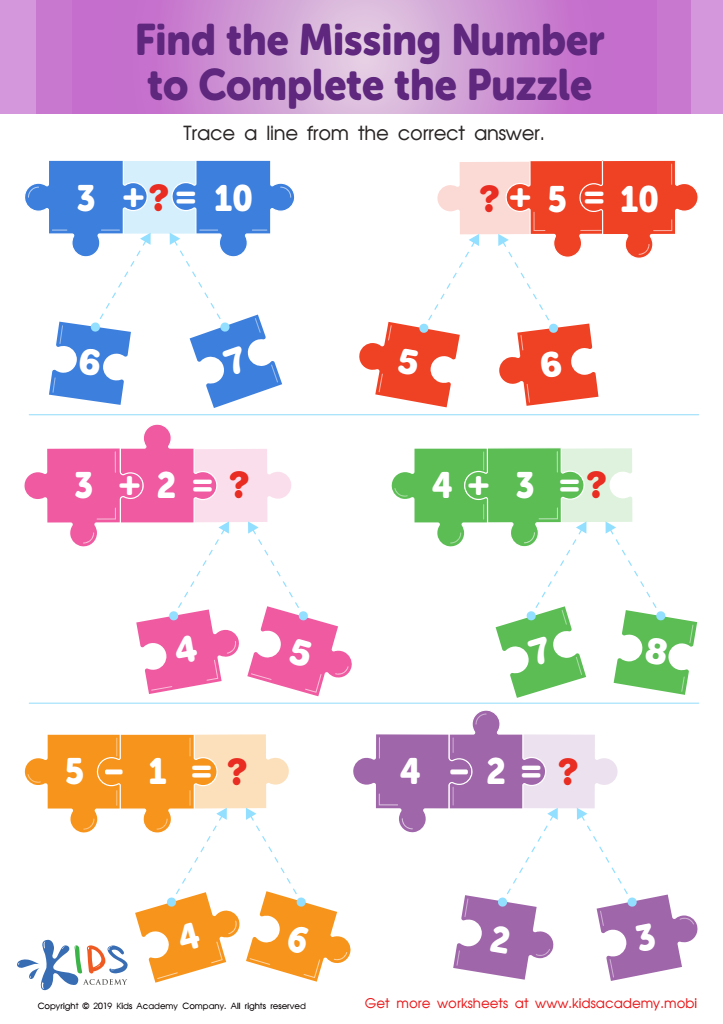Find the Missing Number to Complete the Puzzle Worksheet
Worksheet
Find the Missing Number to Complete the Puzzle Worksheet
Kindergarten
Subtraction
WorksheetFruity Fives! Worksheet
Worksheet
Fruity Fives! Worksheet
In this colorful worksheet, there are three simple equations which your kids must solve. It …
Kindergarten
Subtraction
Worksheet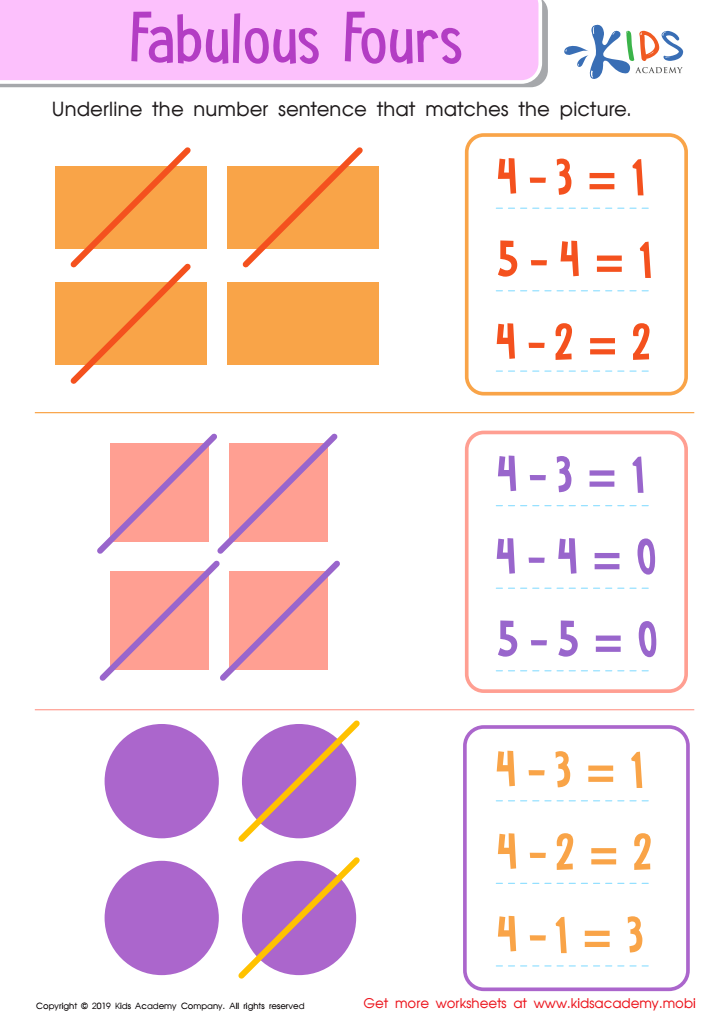Fabulous Fours Worksheet
Worksheet
Fabulous Fours Worksheet
Before your children can go on to more complicated math theories and equations, they must …
Kindergarten
Subtraction
Worksheet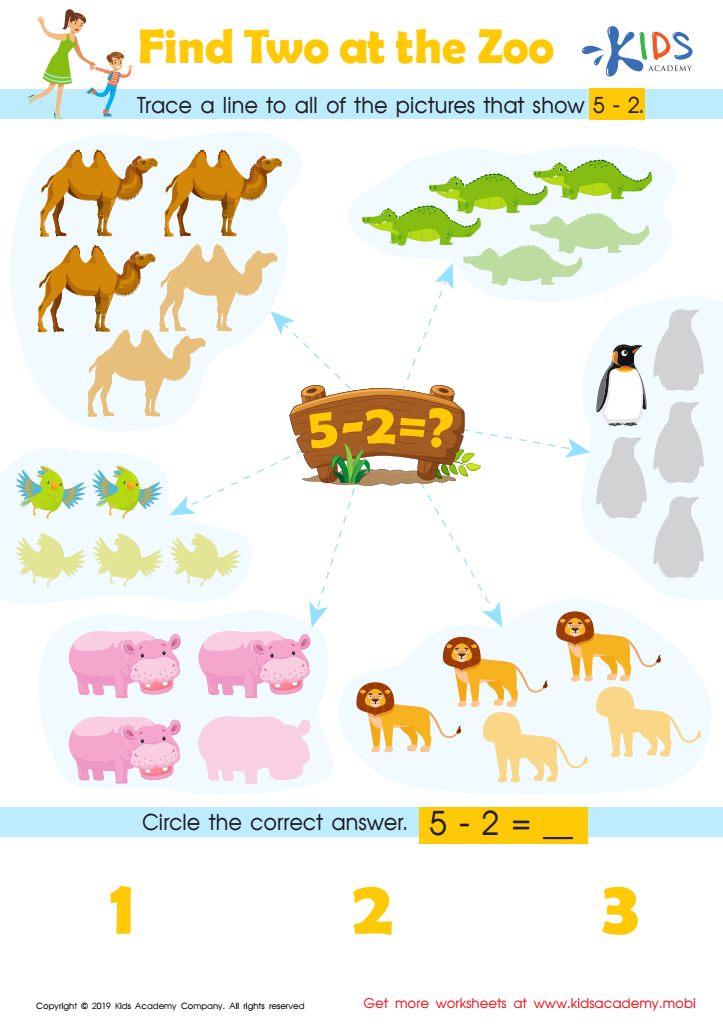Find Two at the Zoo Worksheet
Worksheet
Find Two at the Zoo Worksheet
Kindergarten
Subtraction
Worksheet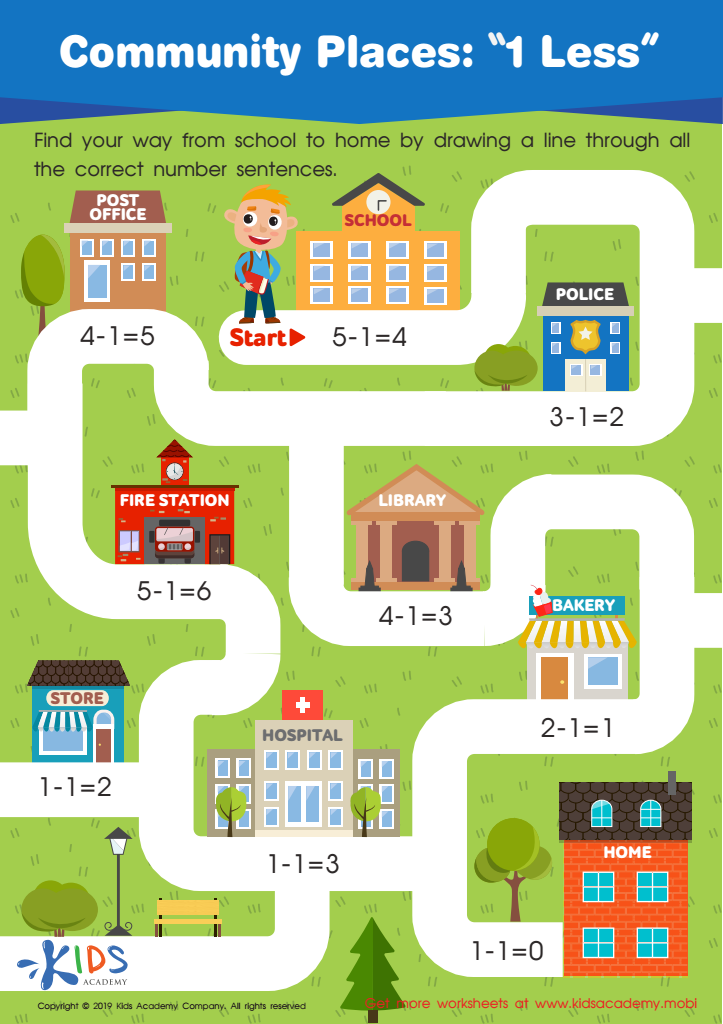Community Places: 1 Less Worksheet
Worksheet
Community Places: 1 Less Worksheet
In a community, there are many different people, places, and buildings. A lot of these …
Kindergarten
Subtraction
Worksheet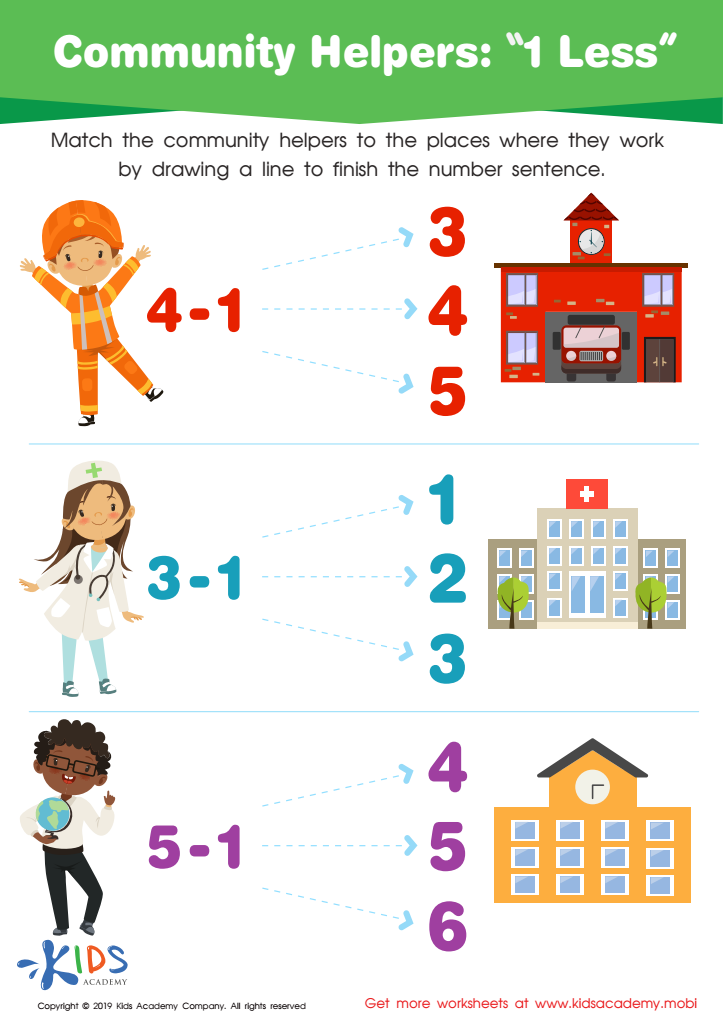Community Helpers: 1 less Worksheet
Worksheet
Community Helpers: 1 less Worksheet
What are some of the things that people do around the community which help the …
Kindergarten
Subtraction
Worksheet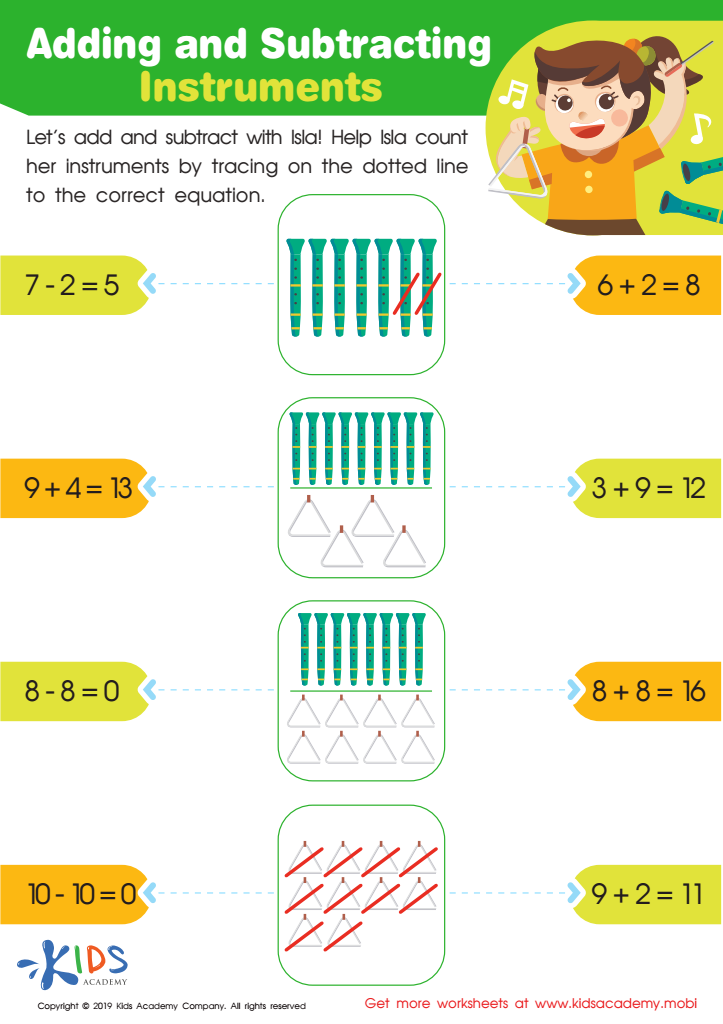Worksheet
This fun worksheet lets children help their friend Isla as she counts the musical instruments …
Subtraction
Worksheet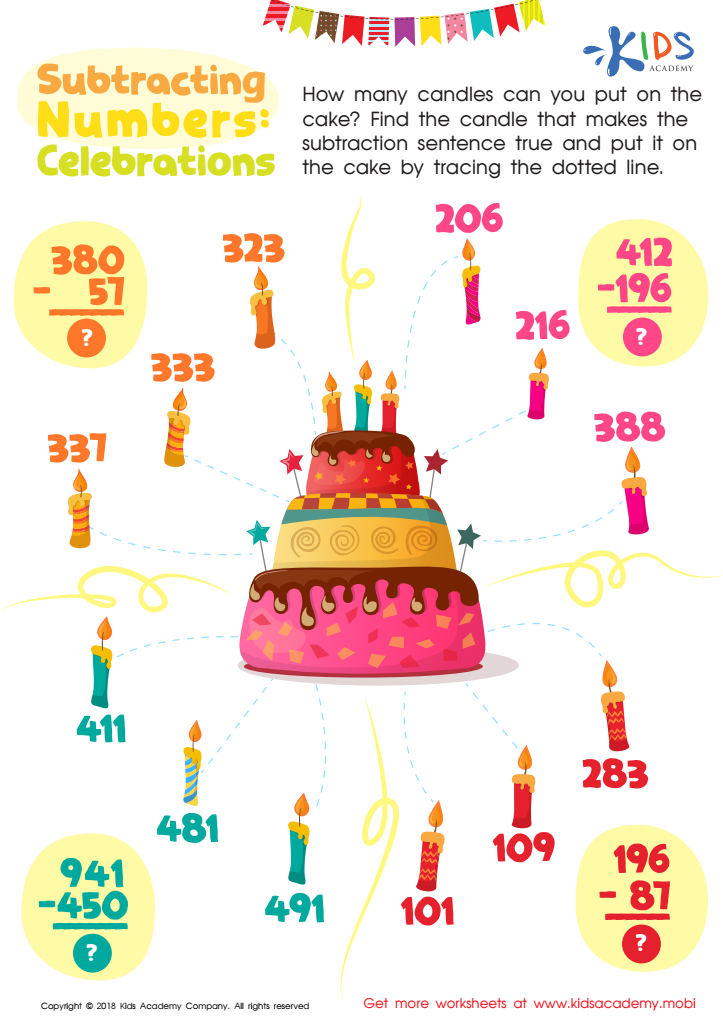Subtracting Numbers: Celebrations Worksheet
Worksheet
Subtracting Numbers: Celebrations Worksheet
Subtraction
Worksheet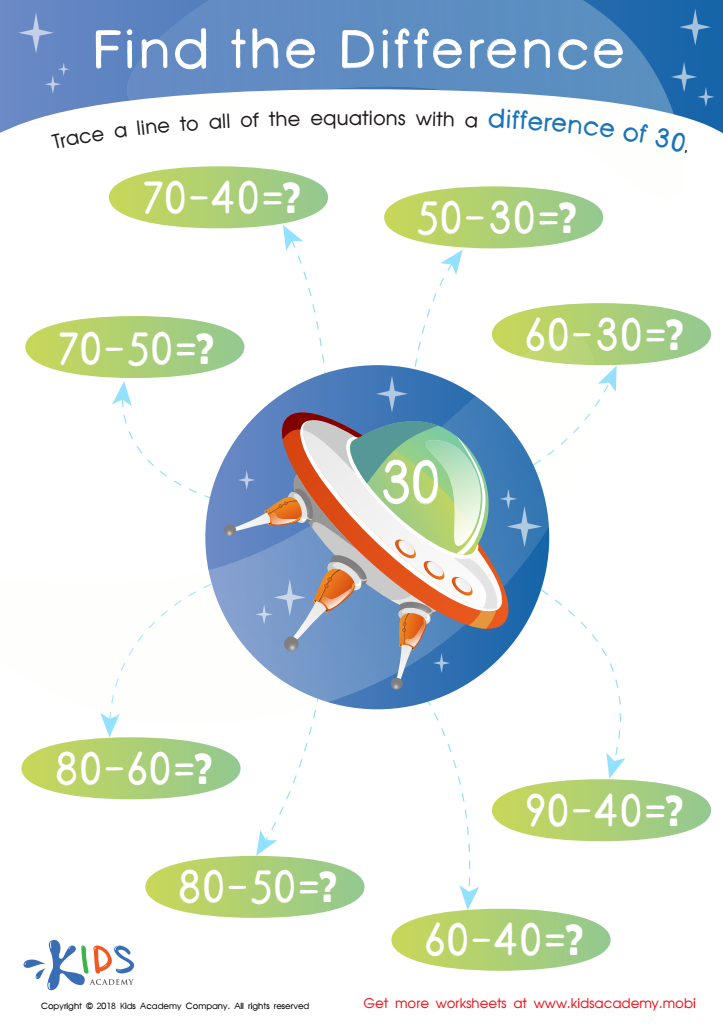Find The Difference Worksheet
Worksheet
Find The Difference Worksheet
Little kindergarteners must be able to correctly solve subtraction problems and arrive at the correct …
Subtraction
Worksheet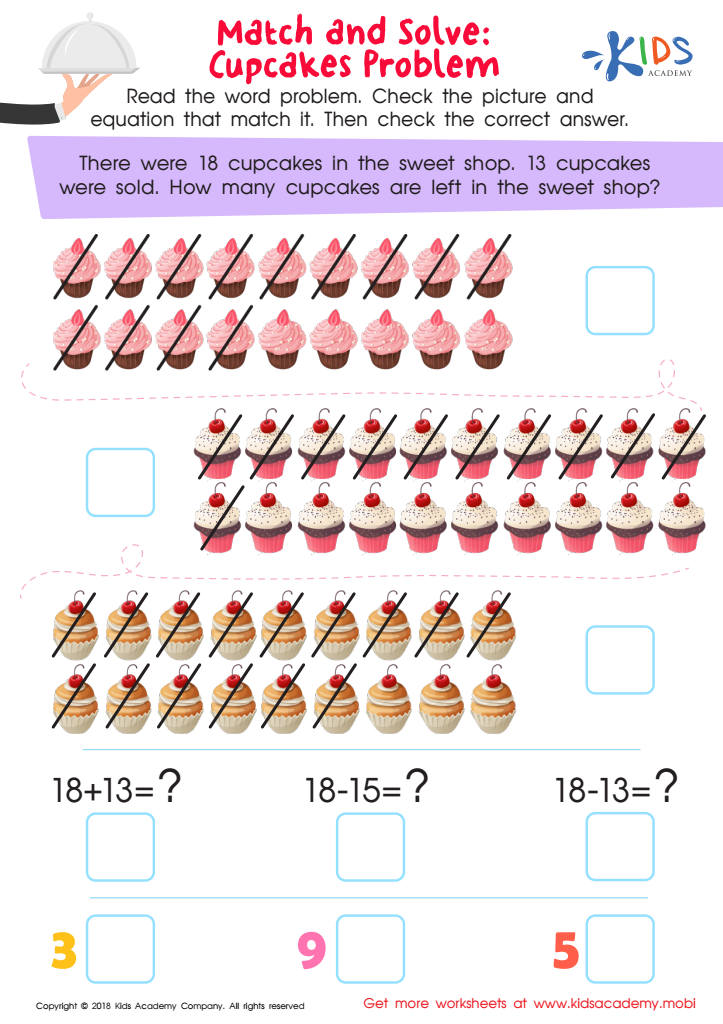Match and Solve: Cupcakes Problem Worksheet
Worksheet
Match and Solve: Cupcakes Problem Worksheet
This colorful math worksheet will tickle your child’s sweet tooth, all while helping him or …
Subtraction
Worksheet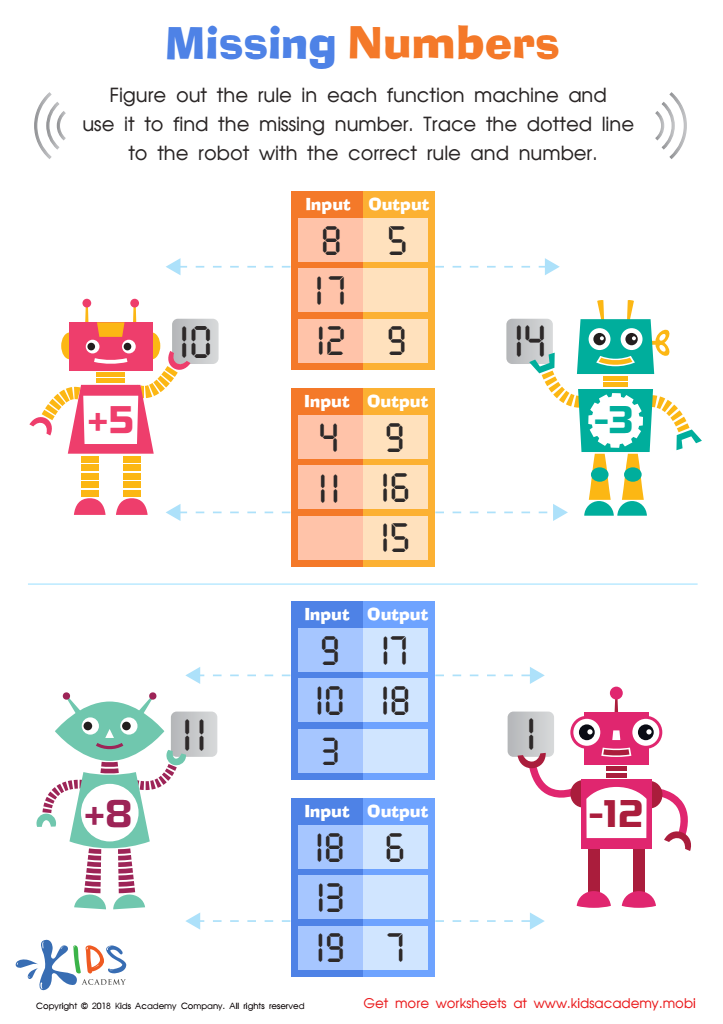Missing Numbers Worksheet
Worksheet
Missing Numbers Worksheet
Solving for missing numbers is a critical pre-algebra skill that even elementary students can learn! …
Subtraction
Worksheet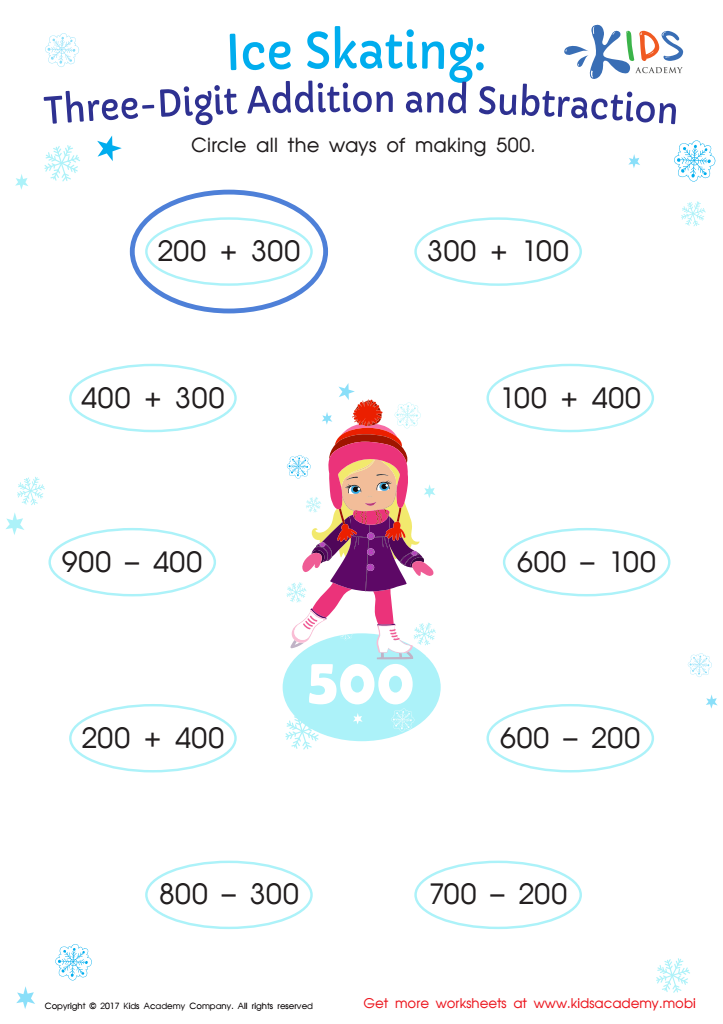Three Digit Addition and Subtraction Worksheet
Worksheet
Three Digit Addition and Subtraction Worksheet
Adding and subtracting with 3 digits can be a tricky task for kids! This 3-digit …
Subtraction
Worksheet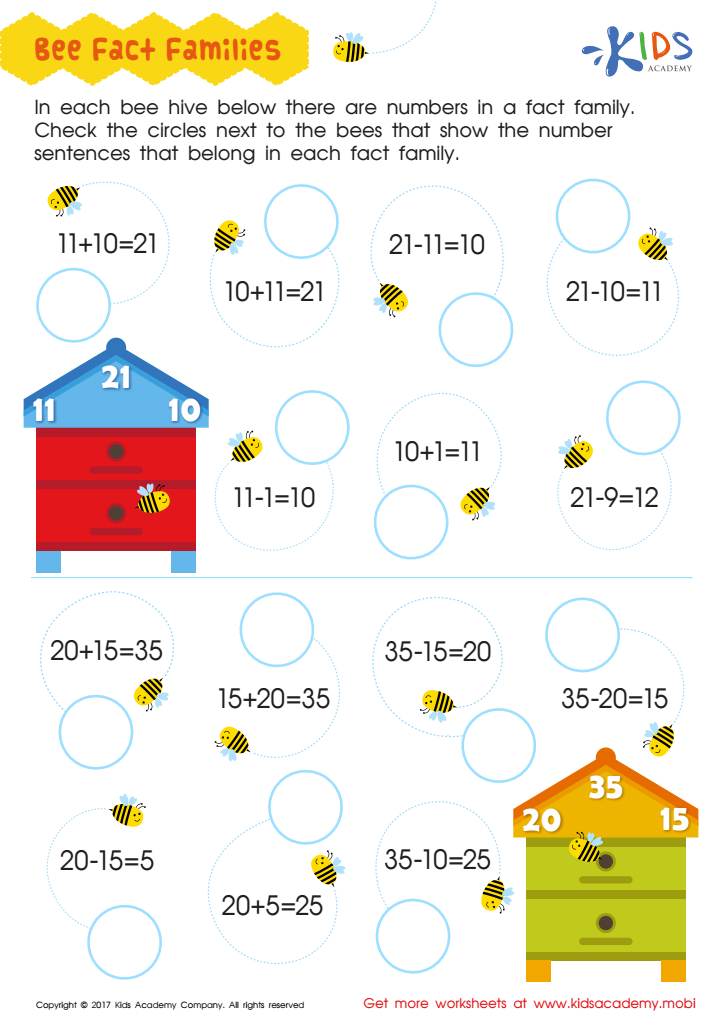Fact Families Worksheet
Worksheet
Fact Families Worksheet
Your child will be buzzing about the beehives on this fun fact families worksheet for …
Subtraction
Worksheet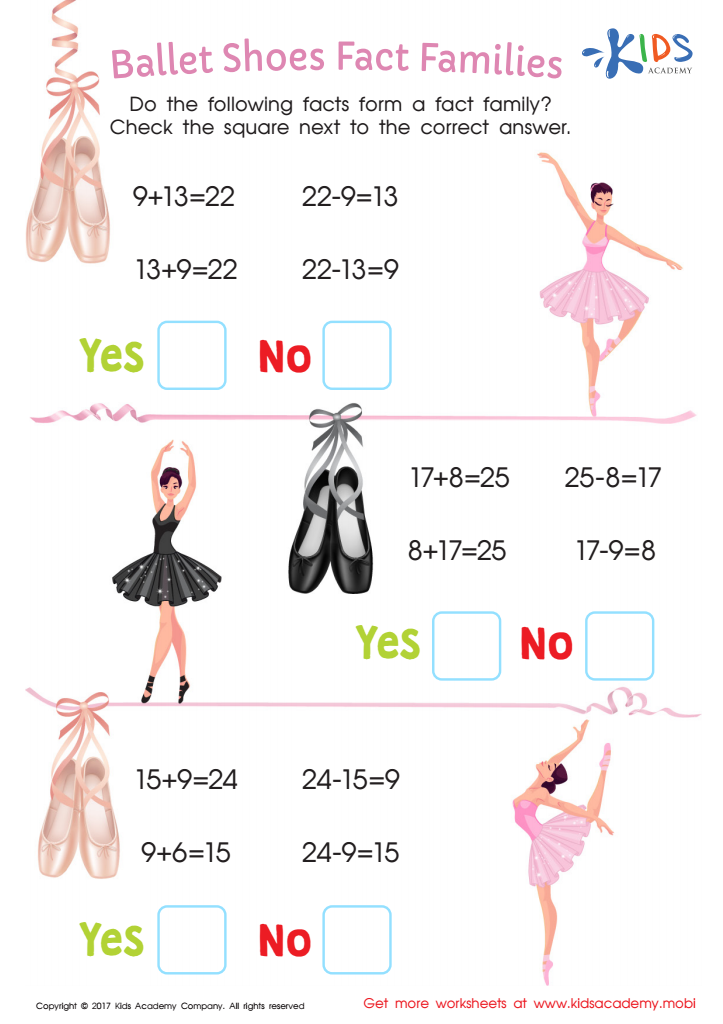Ballet Shoes Fact Family Printable
Worksheet
Ballet Shoes Fact Family Printable
Your child will be delighted to dance her way through this free fact family PDF …
Subtraction
Worksheet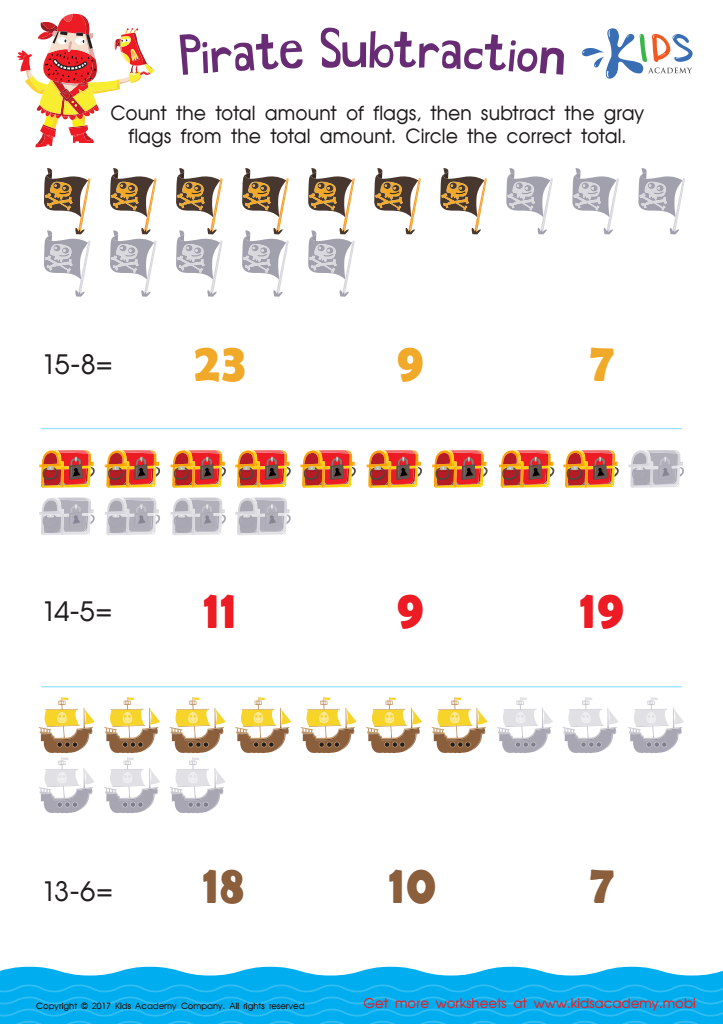Pirate Subtraction Substraction Worksheet
Worksheet
Pirate Subtraction Substraction Worksheet
Ahoy, matey! Let’s learn to subtract the pirate way, with this fun-filled math worksheet: pirate …
Subtraction
Worksheet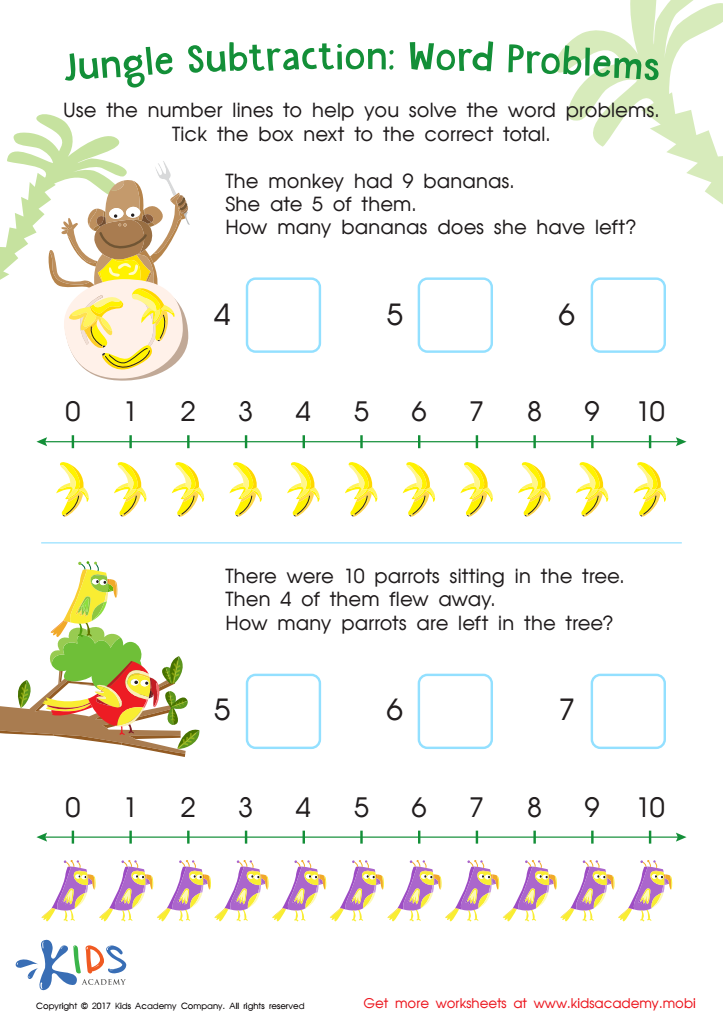Jungle Subtraction Word Problems Substraction Worksheet
Worksheet
Jungle Subtraction Word Problems Substraction Worksheet
Your child will go bananas over this amusing jungle-themed worksheet: subtraction word problems! Read through …
Subtraction
Worksheet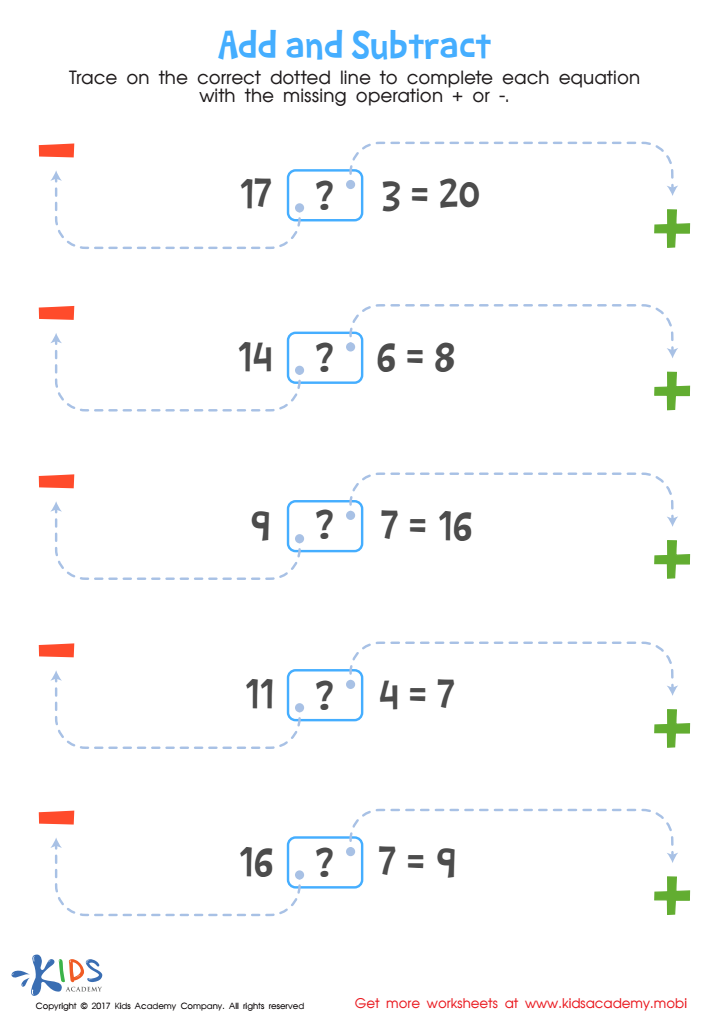Worksheet
As kids learn the basics of math, it’s important to expose them to equations early …
Subtraction
Worksheet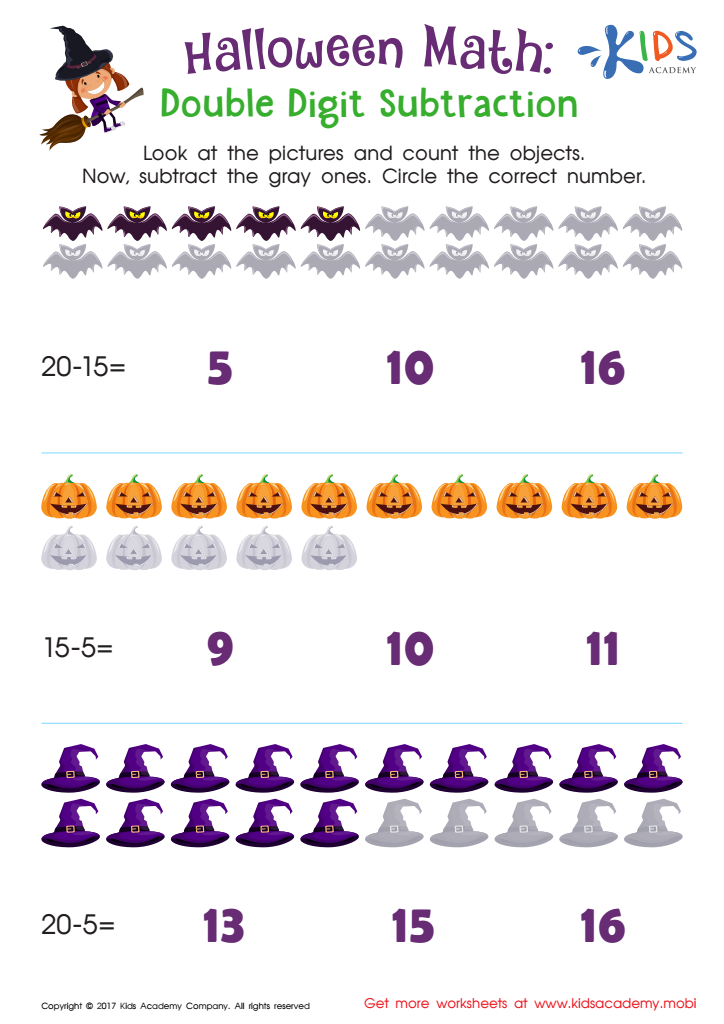Halloween Math Subtraction Worksheet
Worksheet
Halloween Math Subtraction Worksheet
Double digit addition and subtraction can be tricky as numbers get larger and harder to …
Kindergarten
Subtraction
Worksheet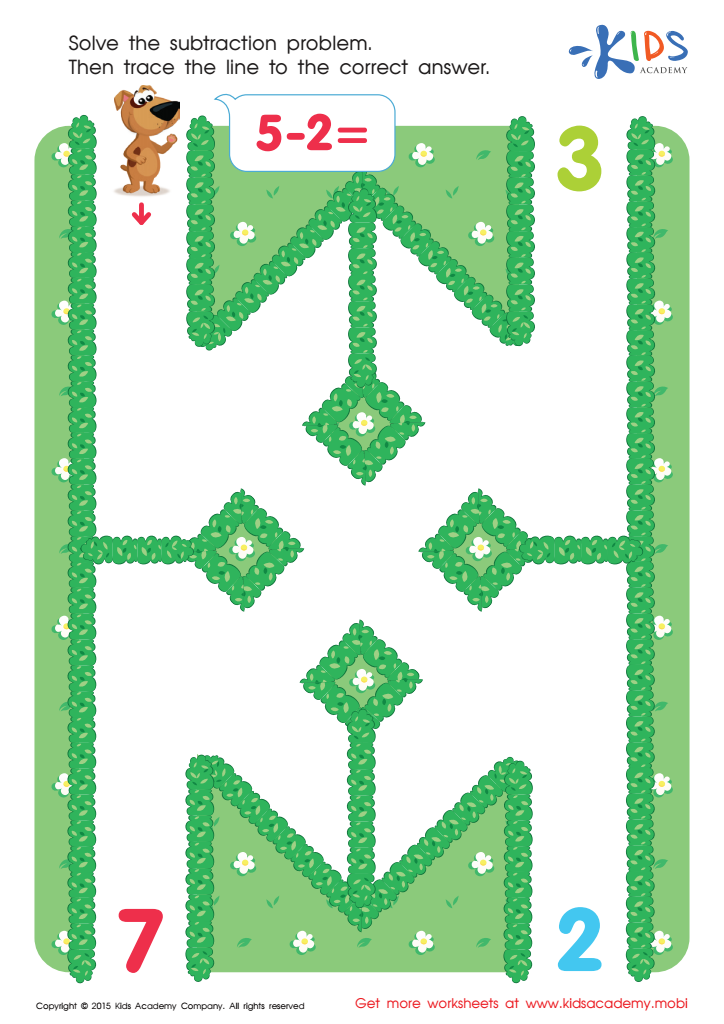Five Minus Two Worksheet
Worksheet
Five Minus Two Worksheet
Math worksheet for kindergarten is nice for practicing subtraction. Studying with Kids Academy is easy …
Kindergarten
Subtraction
Worksheet

Note: You will not be billed until your free trial has ended and can cancel at any time. No strings attached.

Such a great app!
This was a really fun way to get my kids to practice their math. I usually struggle to get them into it and Kids Academy makes it easy.
Fernanndas Stoun
So engaging
We love how we can focus on math with this app. It is engaging and we love how we are able to adjust and tailor the levels according to our son’s knowledge and skill levels!
Momma Anna
Daughter's favorite
Still a top favorite for our 7 year old. She loves earning her stars as she learns to trace letters and numbers. Coloring and the sounds are engaging and are on target for her age group and being Pre K.
Surviving5Kids
Great app
Wow! This app is really great. For the first time my kids actually enjoy learning math and reading. The games on Kids Academy are educational and really fun.
Kimberlyxo
Great app!
This app is wonderful for my 7 years old son! :) It focuses on basic reading, writing, and math skills. The reward system is great and consistent!
Lilbittygarza
Great for kids
Our 7 years old daughter loves reading activities and every off-line game Kids Academy offers. This is a very good app, I highly recommend it!
TonyThan

Extra Challenge worksheets on Subtraction Worksheets can be a great tool for helping children learn to subtract. These worksheets are specifically designed for kids from Pre-K to grade 3 and can help them develop strong math skills and understanding of the concepts of subtraction.

Subtraction can be difficult for some children to learn, but Extra Challenge worksheets help take the frustration and confusion out of the process. By providing an array of subtraction problems that increase in difficulty and challenge the student's math skills, the student will stay engaged while their subtraction ability is being learned and reinforced.

Extra Challenge worksheets use games and visuals to help students learn and understand subtraction. These worksheets provide the opportunity for “hands-on” math learning experiences and make math practice fun!

The use of visuals and drawings make understanding subtraction easier for young learners. By using word problems and stories, students learn how to apply their math skills to solve real-life scenarios. Moreover, the worksheets have been designed specifically for kids from Pre-K to grade 3, helping them to focus on the basics while they develop more complex subtraction strategies.

Each worksheet also includes a section dedicated to explaining common mistakes so that mistakes are clearly noticeable and easy to learn from. This gives students the opportunity to identify and fix mistakes, which helps to build their problem-solving skills and confidence.

Also, the Extra Challenge worksheets provide a wide selection of worksheets with varying difficulty and complexity, which helps to ensure that students remain engaged and challenged while they practice their subtraction. This helps to keet their interest as they progress, keeping them from getting bored with the same type of worksheet.

Overall, Extra Challenge worksheets are extremely useful for helping children learn and master subtraction. They are designed to make understanding and applying subtraction a fun and interesting process. By using different visuals, stories and word problems, students can begin to understand the concepts easily. These worksheets also provide the opportunity to find and fix mistakes, which helps to build a stronger problem-solving ability and confidence. As the student progresses and the worksheets become increasingly difficult, the student remains engaged and challenged. All in all, Extra Challenge worksheets on Subtraction Worksheets are definitely worth considering.